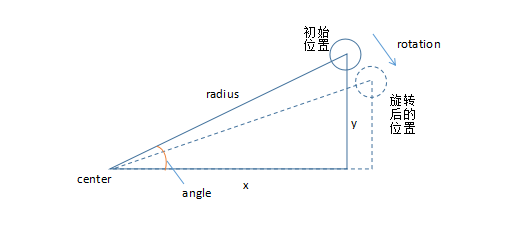# HTML5 Canvas : Advanced Circular Motion

## Review circular motion

The HTML5 Canvas ：Motion And Trajectory paper introduces the realization principle of circular motion, and reviews its calculation method as follows:

``````// (centerx, center) : center coordinates
// angle : the angle of rotation

x =  centerX + Math.sin(angle) * radius
y =  centerY + Math.cos(angle) * radius
``````

The above formula shows that we have known `center point` coordinates, known `radius of rotation` and the angle between the rotating control point and the x-axis, that is, `angle of rotation`. Then, the coordinates of the control points after rotation are calculated based on the rotation radius and the changing rotation angle.

But sometimes...

## When only the coordinates of center point and control point are known

In the past learning process, we know that the distance between two points can be calculated by trigonometric function, which leads to the required radius of rotation, so it can be solved easily:

``````var dx = point.x - center.x,
dy = point.y - center.y,
angle = Math.atan2(dy, dx),
radius = Math.sqrt(dx * dx + dy * dy);
``````

## Only the coordinates of control points and the rotation angle of each frame are known

First, the calculation formula of the control point rotation based on the origin (0, 0) is given

``````// Rotation: angle increment, that is, the angle of rotation based on the initial angle

x = x * Math.cos(rotation) - y * Math.sin(rotation);
y = y * Math.cos(rotation) + x * Math.sin(rotation);
``````

Why do we derive the above formula? The whole derivation process is as follows:1. As can be seen from the above figure, the coordinates of control points can be calculated as follows:
``````x = radius * cos(angle);
``````
1. After rotation, the coordinates of the control points can be predicted as follows:
``````// angle : initial angle, that is, the angle between the known point at the initial position and the x-axis
// rotation : angle increment, that is, the angle of rotation based on the initial angle

x = radius * cos(angle + rotation);
y = raidus * sin(angle + rotation);
``````
1. We know that trigonometric functions contain some common induction formulas, which need to be used here:
``````cos(a + b) = cos(a) * cos(b) - sin(a) * sin(b);
sin(a + b) = sin(a) * cos(b) + cos(a) * sin(b);
``````
1. Then, expand the predictive coordinate formula of the control point:
``````x = radius * cos(angle) * cos(rotation) - raidus * sin(angle) * sin(rotation);
y = raidus * sin(angle) * cos(rotation) + raidus * cos(angle) * sin(rotation);
``````
1. Eliminate the superfluous items and come to the conclusion:
``````/ / because
// x = radius * cos(angle);
// y = radius * sin(angle);
// By substituting it, we can get:

x = x * Math.cos(rotation) - y * Math.sin(rotation);
y = y * Math.cos(rotation) + x * Math.sin(rotation);
``````
1. The previous calculation can be regarded as rotating around the origin (0, 0), so when we can place the center point at any point in the coordinate system, we can get the formula:
``````x = center.x + (x - center.x) * cos(rotation) - (y - center.y) * sin(rotation);
y = center.y + (y - center.y) * cos(rotation) + (x - center.x) * sin(rotation);
``````

It is easy to understand. We can imagine that the known coordinates of the center point are regarded as the origin, and after the control point rotates, plus the x-axis and y-axis distance between the center point and the real origin to return to the real coordinate system.

Here's a chestnut:

``````<html>
<body>
<canvas id="myCanvas" width="200" height="200" style="border: 1px solid">
</canvas>
</body>
</html>
<script>
(function () {
// canvas
var canvas = document.getElementById("myCanvas");
var ctx = canvas.getContext("2d");

// known center points and control points rotating around them
var center = { x: 100, y: 100 };
var point = { x: 50, y: 100 };

// that the unframe control point rotates 30 degrees based on the angle of the previous frame
var rotation = 30 * Math.PI / 180;

setInterval(function () {
ctx.clearRect(0, 0, 200, 200);

var x = center.x + (point.x - center.x) * Math.cos(rotation) - (point.y - center.y) * Math.sin(rotation);
var y = center.y + (point.y - center.y) * Math.cos(rotation) + (point.x - center.x) * Math.sin(rotation);

point.x = x
point.y = y

// draw a ball
ctx.beginPath();
ctx.arc(point.x, point.y, 10, 0, 2 * Math.PI);
ctx.fill();

/ / painting center
ctx.beginPath();
ctx.arc(center.x, center.y, 2, 0, 2 * Math.PI, true);
ctx.fill();

}, 100)
})()
</script>
``````